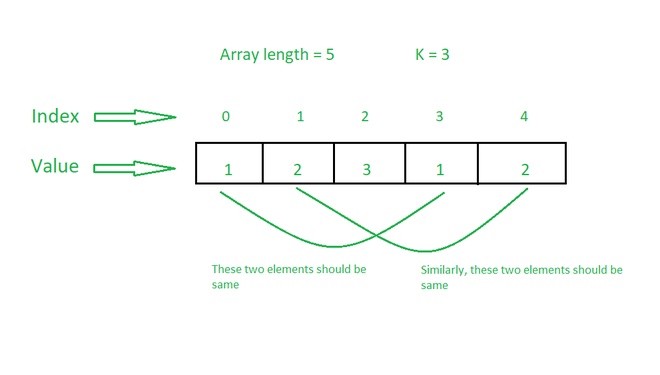# Minimum replacements required to make sum of all K-length subarrays equal

• Difficulty Level : Medium
• Last Updated : 13 Jun, 2022

Given an array arr[] consisting of N positive integers and an integer K, the task is to make the sum of all K-length subarrays equal by replacing minimum number of array elements with any integer.

Examples:

Input: arr[] = {3, 4, 3, 5, 6}, K = 2
Output: 2
Explanation:
Operation 1: Replacing arr by 4 modifies arr[] to {3, 4, 3, 4, 6}.
Operation 2: Replacing arr by 3 modifies arr[] to {3, 4, 3, 4, 3}.
All subarrays of length 2 are {{3, 4}, {4, 3}, {3, 4}, {4, 3}}. Sum of all these subarrays is 7. Therefore, the minimum number of operations required is 2.

Input: arr[] = {1, 2, 3, 1, 2}, K = 3
Output: 0
Explanation: All subarrays of length 3 are {{1, 2, 3}, {2, 3, 1}, {3, 1, 2}}. Since all these subarrays have sum 6, the number of operations required is 0.

Approach: The idea is based on the observation that all subarrays will have equal sum, when all elements separated by distance K are equal.Therefore, the problem can be solved by counting the frequency of elements separated by a distance K and find the number which appears maximum times. Follow the steps below to solve the problem:

• Initialize a variable ans to store the required result.
• Iterate in the range [0, K-1] using the variable i
• Create a map, freq to store the frequency of elements separated by a distance K starting from i.
• Traverse the map and find the element which occurs the maximum number of times.
• Again, traverse the map and if the element is not equal to the maximum occurring element then add its frequency to the ans.
• Print the value of ans as the result.

Below is the implementation of the above approach:

## C++

 `// C++ program for the above approach``#include ``using` `namespace` `std;` `// Function to find minimum number of``// operations required to make sum of``// all subarrays of size K equal``void` `findMinOperations(``int` `arr[],``                       ``int` `N, ``int` `K)``{``    ``// Stores number of operations``    ``int` `operations = 0;` `    ``// Iterate in the range [0, K-1]``    ``for` `(``int` `i = 0; i < K; i++) {` `        ``// Stores frequency of elements``        ``// separated by distance K``        ``unordered_map<``int``, ``int``> freq;` `        ``for` `(``int` `j = i; j < N; j += K)``            ``freq[arr[j]]++;` `        ``// Stores maximum frequency``        ``// and corresponding element``        ``int` `max1 = 0, num;` `        ``// Find max frequency element``        ``// and its frequency``        ``for` `(``auto` `x : freq) {``            ``if` `(x.second > max1) {``                ``max1 = x.second;``                ``num = x.first;``            ``}``        ``}` `        ``// Update the number of operations``        ``for` `(``auto` `x : freq) {``            ``if` `(x.first != num)``                ``operations += x.second;``        ``}``    ``}` `    ``// Print the result``    ``cout << operations;``}` `// Driver Code``int` `main()``{``    ``// Given Input``    ``int` `arr[] = { 3, 4, 3, 5, 6 };``    ``int` `K = 2;``    ``int` `N = ``sizeof``(arr) / ``sizeof``(arr);` `    ``// Function Call``    ``findMinOperations(arr, N, K);` `    ``return` `0;``}`

## Java

 `// Java program for the above approach``import` `java.lang.*;``import` `java.util.*;` `class` `GFG``{``  ` `  ``// Function to find minimum number of``  ``// operations required to make sum of``  ``// all subarrays of size K equal``  ``static` `void` `findMinOperations(``int` `arr[],``                                ``int` `N, ``int` `K)``  ``{` `    ``// Stores number of operations``    ``int` `operations = ``0``;` `    ``// Iterate in the range [0, K-1]``    ``for` `(``int` `i = ``0``; i < K; i++) {` `      ``// Stores frequency of elements``      ``// separated by distance K``      ``Map freq=``new` `HashMap<>();` `      ``for` `(``int` `j = i; j < N; j += K)``        ``freq.put(arr[j], freq.getOrDefault(arr[j],``0``)+``1``);` `      ``// Stores maximum frequency``      ``// and corresponding element``      ``int` `max1 = ``0``, num=-``1``;` `      ``// Find max frequency element``      ``// and its frequency``      ``for` `(Map.Entry x : freq.entrySet()) {``        ``if` `(x.getValue() > max1) {``          ``max1 = x.getValue();``          ``num = x.getKey();``        ``}``      ``}` `      ``// Update the number of operations``      ``for` `( Map.Entry x : freq.entrySet()) {``        ``if` `(x.getKey() != num)``          ``operations += x.getValue();``      ``}``    ``}` `    ``// Print the result``    ``System.out.print(operations);``  ``}` `  ``// Driver code``  ``public` `static` `void` `main(String[] args)``  ``{` `    ``// Given Input``    ``int` `arr[] = { ``3``, ``4``, ``3``, ``5``, ``6` `};``    ``int` `K = ``2``;``    ``int` `N = arr.length;` `    ``// Function Call``    ``findMinOperations(arr, N, K);``  ``}` `}` `// This code is contributed by offbeat`

## Python3

 `# python 3 program for the above approach` `# Function to find minimum number of``# operations required to make sum of``# all subarrays of size K equal``def` `findMinOperations(arr, N, K):``    ``# Stores number of operations``    ``operations ``=` `0` `    ``# Iterate in the range [0, K-1]``    ``for` `i ``in` `range``(K):``        ``# Stores frequency of elements``        ``# separated by distance K``        ``freq ``=` `{}` `        ``for` `j ``in` `range``(i,N,K):``            ``if` `arr[j] ``in` `freq:``                ``freq[arr[j]] ``+``=` `1``            ``else``:``                ``freq[arr[j]] ``=` `1` `        ``# Stores maximum frequency``        ``# and corresponding element``        ``max1 ``=` `0``        ``num ``=` `0` `        ``# Find max frequency element``        ``# and its frequency``        ``for` `key,value ``in` `freq.items():``            ``if` `(value > max1):``                ``max1 ``=` `value``                ``num ``=` `key` `        ``# Update the number of operations``        ``for` `key,value ``in` `freq.items():``            ``if` `(key !``=` `num):``                ``operations ``+``=` `value` `    ``# Print the result``    ``print``(operations)` `# Driver Code``if` `__name__ ``=``=` `'__main__'``:``  ` `    ``# Given Input``    ``arr ``=` `[``3``, ``4``, ``3``, ``5``, ``6``]``    ``K ``=` `2``    ``N ``=` `len``(arr)` `    ``# Function Call``    ``findMinOperations(arr, N, K)``    ` `    ``# This code is contributed by ipg2016107.`

## C#

 `// C# program for the above approach``using` `System;``using` `System.Collections.Generic;` `class` `GFG{`` ` `// Function to find minimum number of``// operations required to make sum of``// all subarrays of size K equal``static` `void` `findMinOperations(``int` `[]arr,``                              ``int` `N, ``int` `K)``{``    ` `    ``// Stores number of operations``    ``int` `operations = -1;` `    ``// Iterate in the range [0, K-1]``    ``for``(``int` `i = 0; i < K; i++)``    ``{``        ` `        ``// Stores frequency of elements``        ``// separated by distance K``        ``Dictionary<``int``,``                   ``int``> freq = ``new` `Dictionary<``int``,``                                              ``int``>();``                                              ` `        ``for``(``int` `j = i; j < N; j += K)``        ``{``            ``if` `(freq.ContainsKey(arr[j]))``                ``freq[arr[j]]++;``            ``else``                ``freq.Add(arr[j], 1);``        ``}` `        ``// Stores maximum frequency``        ``// and corresponding element``        ``int` `max1 = -1, num = 0;` `        ``// Find max frequency element``        ``// and its frequency``        ``foreach``(KeyValuePair<``int``, ``int``> entry ``in` `freq)``        ``{``            ``if` `(entry.Key > max1)``            ``{``                ``max1 = entry.Value;``                ``num = entry.Key;``            ``}``        ``}``    ` `        ``// Update the number of operations``        ``foreach``(KeyValuePair<``int``, ``int``> entry ``in` `freq)``        ``{``            ``if` `(entry.Key != num)``                ``operations += entry.Value;``        ``}``    ``}` `    ``// Print the result``    ``Console.Write(operations);``}` `// Driver Code``public` `static` `void` `Main()``{``    ` `    ``// Given Input``    ``int` `[]arr = { 3, 4, 3, 5, 6 };``    ``int` `K = 2;``    ``int` `N = arr.Length;` `    ``// Function Call``    ``findMinOperations(arr, N, K);``}``}` `// This code is contributed by SURENDRA_GANGWAR`

## Javascript

 ``

Output:

`2`

Time Complexity: O(N)
Auxiliary Space: O(N)

My Personal Notes arrow_drop_up